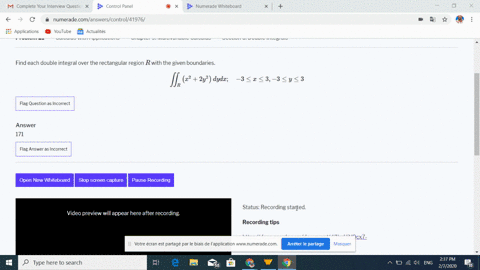🎉 The Study-to-Win Winning Ticket number has been announced! Go to your Tickets dashboard to see if you won! 🎉View Winning Ticket### Find each double integral over the rectangular re…

11:00Georgia Southern University
Problem 20

# Evaluate each iterated integral. (Many of these use results from Exercises 1-10 ). $$\int_{3}^{4} \int_{1}^{2}\left(\frac{6 x}{5}+\frac{y}{x}\right) d x d y$$

## Discussion

You must be signed in to discuss.

## Video Transcript

Okay, so here we have this double inner girl. Three to four and two of the function, six x over five. Plus, why ever X And that's the extra y. So first, we want to take an anti derivative here with respect to X keeping. Why fixed? So that ends up being three x squared over five. I mean, that x x squared over two. Plus, why long absolute value of X evaluated from one to in that function of libel, integrate with respected by. Okay, so we can just evaluate the's in points x squared. So we force this is going to be three fifths. Two squared, minus one squared. Plus why? Times Law of two minds Log of Warren. Remember that log of one zero? Okay, do you want? So this is just the inner girl from three to four. So you're going to be three. So that will be nine fifths. Plus. Why? Log of two? It's like one zero. Okay, now, just taken an anti derivative with respect to why said nine fifths. Why? Plus why squared over two? Walk to how you waited from three to four. Could appear. Okay, so then if I evaluate Why? From three to four that just gives me once I get nine, Sure. And then why squared? Evaluated from four squared minus three squared? That's sixteen a minus nine. That seven. So seven over two look two.